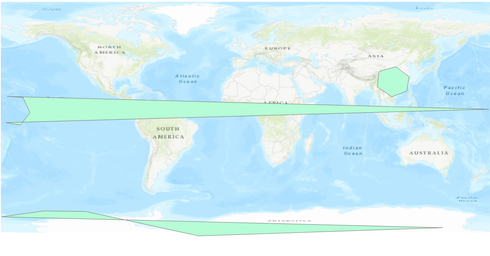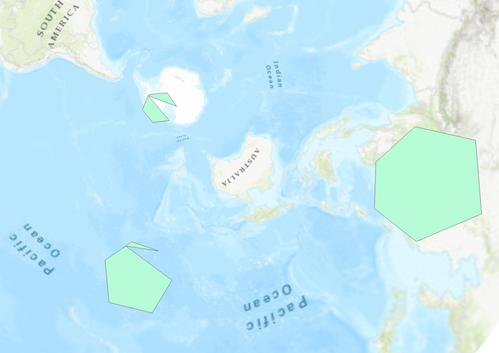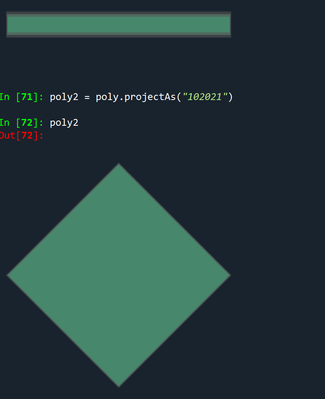# Polygon geometry at antimeridian and poles not drawing correctly

921
1
02-28-2021 04:21 PMNew Contributor III

Hello,

I noticed an issue with creating polygons at the antimeridian and poles, and was hoping someone could weigh in.

Running the below code will create 3 polys, one a normal one to show the geometry we'd expect, one at the antimeridian and one at the south pole.

The antimeridian polygon has stretched itself around the world because the longitude varies from -175 to -179. I can work around this by looking at the min and max longitude, and if they're >180 degrees then add 360 degrees to the negative longitudes. There has to be a better way to do this than that though??

For southpole, the polygon is distorted when viewing in WGS84 which is expected. However changing it to South Pole Stereographic, the polygon still doesn't look as it should.

While diagnosing why the polygon wasn't drawing correctly (it should look like 'normal' in southpole stereographic), I extracted the polygons from the featureclass. It goes into the featureclass with 7 points (including the same start and end point), and comes out with 10, with the start and end different? (the print out of the coordinates is at the bottom of this post).

What is going on here?

``````import arcpy
WGS84 = arcpy.SpatialReference('WGS 1984')

'''Add a list of points as a polygon to a fc'''

arr = arcpy.Array([arcpy.Point(*coords) for coords in points])
poly = arcpy.Polygon(arr, WGS84)

with arcpy.da.InsertCursor(fc, ['name', 'shape@']) as ic:
ic.insertRow([name, poly])

fc = arcpy.management.CreateFeatureclass(r"D:\testing\unusual_polygons.gdb",
'Poly', 'POLYGON', spatial_reference=WGS84).getOutput(0)

normal = ((118.80300158646772, 19.252194904738648),
(120.50339385995798, 29.892985338693542),
(110.69461054882274, 37.16389648579593),
(98.22850425577053, 33.6210697806835),
(97.14850212623087, 22.29703635237555),
(107.74262433198103, 15.063301114735607),
(118.80300148646772, 19.252194904738648))

southpole = ((-179.67438964805672, -73.31022368544396),
(145.24158201971542, -81.29137179020501),
(-34.441802308663334, -87.36469532319619),
(-85.85690989815225, -76.16304283019099),
(-117.65345504349024, -69.3935964899183),
(-148.16871950091263, -68.92995788193983),
(-179.67438964805672, -73.31022368544396))
antimeridian = ((-175.45827111402537, 15.285730312483953),
(179.2171608248945, 5.8899217543138915),
(-176.05696384421353, -3.968796976609585),
(-165.41674992858833, -5.7628604914369195),
(-158.59967491814012, 3.3359348575173495),
(-163.71455965998953, 14.623074892227512),
(-175.45827111402537, 15.285730312483953))

# Also print the length of each polygon (7 vertices long. This is important)
for name, p in [('normal', normal), ('southpole', southpole), ('antimeridian', antimeridian)]:
print(name, len(p))

# Now print the length of each polygon. The polygons started with 7 points, and now they have 10?
with arcpy.da.SearchCursor(fc, ['name', 'shape@']) as sc:
for row in sc:
poly = row
print(name, repr(poly), poly.pointCount)
for part in poly:
for point in part:
print(f'    {point.X:19.17} {point.Y:19.17}')
print()``````

The polygons in WG84.In polar stereographic. Pole and antimerdian polygons not drawing correctly.The coords after being added to the featureclass:

```normal <Polygon object at 0x2511ff727b8[0x25125b18c38]> 8
118.80300158600005  19.252194905000067
118.80300148600008  19.252194905000067
107.74262433200005  15.063301115000058
97.148502126000039  22.297036352000077
98.228504256000065  33.621069781000074
110.69461054900006  37.163896486000056
120.50339386000007  29.892985339000063
118.80300158600005  19.252194905000067

southpole <Polygon object at 0x2511fc03198[0x2511f66d058]> 10
-88.768273812999951 -75.543216942999948
145.24158202000001 -81.291371789999971
-34.441802308999968 -87.364695322999978
-85.856909897999969 -76.163042829999938
-88.768273812999951 -75.543216942999948
-179.67438964799999 -73.310223684999983
-148.16871950099997  -68.92995788199994
-117.65345504299995 -69.393596489999936
-88.768273812999951 -75.543216942999948
-179.67438964799999 -73.310223684999983

antimeridian <Polygon object at 0x2511ff727b8[0x25125b18c38]> 10
-163.81813172799997 -3.6291741479999473
-165.41674992899996 -5.7628604909999694
-176.05696384399997  -3.968796976999954
-163.81813172799997 -3.6291741479999473
-175.45827111399998  15.285730312000055
179.21716082500006  5.8899217540000564
-163.81813172799997 -3.6291741479999473
-158.59967491799998  3.3359348580000301
-163.71455965999996  14.623074892000034
-175.45827111399998  15.285730312000055```

I have tried adding just the south pole poly to a south pole stereographic projected featureclass to see if it's a projection problem, but I get the same output. I tried also using the defense mapping tool 'coordinate table to polygon' but again same issue.

Thanks 🙂

Tags (4)byMVP Esteemed Contributor

I will just do an example in python arcpy... note the replication on either side of the meridian

``````p = np.array([[-180.,-90.], [-180., -80.], [-90., -80.], [0., -80.],
[90., -80.], [180., -80.], [180., -90.]])

aa = [Point(*pairs) for pairs in p]
poly = Polygon(Array(aa), "4326")
poly2 = poly.projectAs("102021")``````... sort of retired...